## Interference

7-29-99

### The wave nature of light

When we discussed the reflection and refraction of light, light was interacting with mirrors and lenses. These objects are much larger than the wavelength of light, so the analysis can be done using geometrical optics, a simple model that uses rays and wave fronts. In this chapter we'll need a more sophisticated model, physical optics, which treats light as a wave. The wave properties of light are important in understanding how light interacts with objects such as narrow openings or thin films that are about the size of the wavelength of light.

Because physical optics deals with light as a wave, it is helpful to have a quick review of waves. The principle of linear superposition is particularly important.

### Linear superposition

When two or more waves come together, they will interfere with each other. This interference may be constructive or destructive. If you take two waves and bring them together, they will add wherever a peak from one matches a peak from the other. That's constructive interference. Wherever a peak from one wave matches a trough in another wave, however, they will cancel each other out (or partially cancel, if the amplitudes are different); that's destructive interference.

The most interesting cases of interference usually involve identical waves, with the same amplitude and wavelength, coming together. Consider the case of just two waves, although we can generalize to more than two. If these two waves come from the same source, or from sources that are emitting waves in phase, then the waves will interfere constructively at a certain point if the distance traveled by one wave is the same as, or differs by an integral number of wavelengths from, the path length traveled by the second wave. For the waves to interfere destructively, the path lengths must differ by an integral number of wavelengths plus half a wavelength.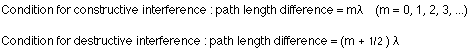### Young's double slit

Light, because of its wave properties, will show constructive and destructive interference. This was first shown in 1801 by Thomas Young, who sent sunlight through two narrow slits and showed that an interference pattern could be seen on a screen placed behind the two slits. The interference pattern was a set of alternating bright and dark lines, corresponding to where the light from one slit was alternately constructively and destructively interfering with the light from the second slit.

You might think it would be easier to simply set up two light sources and look at their interference pattern, but the phase relationship between the waves is critically important, and two sources tend to have a randomly varying phase relationship. With a single source shining on two slits, the relative phase of the light emitted from the two slits is kept constant.

This makes use of Huygen's principle, the idea that each point on a wave can be considered to be a source of secondary waves. Applying this to the two slits, each slit acts as a source of light of the same wavelength, with the light from the two slits interfering constructively or destructively to produce an interference pattern of bright and dark lines.This pattern of bright and dark lines is known as a fringe pattern, and is easy to see on a screen. The bright fringe in the middle is caused by light from the two slits traveling the same distance to the screen; this is known as the zero-order fringe.The dark fringes on either side of the zero-order fringe are caused by light from one slit traveling half a wavelength further than light from the other slit. These are followed by the first-order fringes (one on each side of the zero-order fringe), caused by light from one slit traveling a wavelength further than light from the other slit, and so on.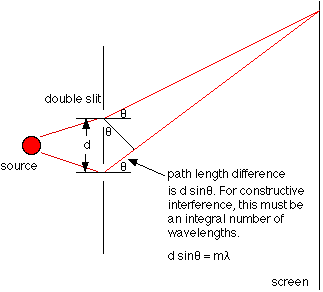The diagram above shows the geometry for the fringe pattern. For two slits separated by a distance d, and emitting light at a particular wavelength, light will constructively interfere at certain angles. These angles are found by applying the condition for constructive interference, which in this case becomes: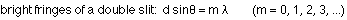The angles at which dark fringes occur can be found be applying the condition for destructive interference: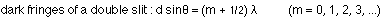If the interference pattern was being viewed on a screen a distance L from the slits, the wavelength can be found from the equation: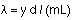where y is the distance from the center of the interference pattern to the mth bright line in the pattern. That applies as long as the angle is small (i.e., y must be small compared to L).

### Dispersion

Although we talk about an index of refraction for a particular material, that is really an average value. The index of refraction actually depends on the frequency of light (or, equivalently, the wavelength). For visible light, light of different colors means light of different wavelength. Red light has a wavelength of about 700 nm, while violet, at the other end of the visible spectrum, has a wavelength of about 400 nm.

This doesn't mean that all violet light is at 400 nm. There are different shades of violet, so violet light actually covers a range of wavelengths near 400 nm. Likewise, all the different shades of red light cover a range near 700 nm.

Because the refractive index depends on the wavelength, light of different colors (i.e., wavelengths) travels at different speeds in a particular material, so they will be refracted through slightly different angles inside the material. This is called dispersion, because light is dispersed into colors by the material.

When you see a rainbow in the sky, you're seeing something produced by dispersion and internal reflection of light in water droplets in the atmosphere. Light from the sun enters a spherical raindrop, and the different colors are refracted at different angles, reflected off the back of the drop, and then bent again when they emerge from the drop. The different colors, which were all combined in white light, are now dispersed and travel in slightly different directions. You see red light coming from water droplets higher in the sky than violet light. The other colors are found between these, making a rainbow.

Rainbows are usually seen as half circles. If you were in a plane or on a very tall building or mountain, however, you could see a complete circle. In double rainbows the second, dimmer, band, which is higher in the sky than the first, comes from light reflected twice inside a raindrop. This reverses the order of the colors in the second band.